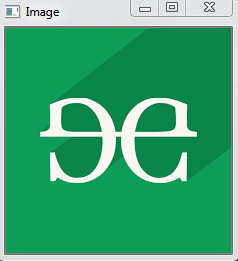# Python OpenCV – cv2.flip() method

• Last Updated : 08 Jul, 2020

OpenCV-Python is a library of programming functions mainly aimed at real-time computer vision. `cv2.flip()` method is used to flip a 2D array. The function cv::flip flips a 2D array around vertical, horizontal, or both axes.

Syntax: cv2.cv.flip(src, flipCode[, dst] )

Parameters:
src: Input array.
dst: Output array of the same size and type as src.
flip code: A flag to specify how to flip the array; 0 means flipping around the x-axis and positive value (for example, 1) means flipping around y-axis. Negative value (for example, -1) means flipping around both axes.

Return Value: It returns an image.

Image used for all the below examples:Example:

 `# Python program to explain cv2.flip() method`` ` `# importing cv2``import` `cv2`` ` `# path``path ``=` `r``'C:\Users\user\Desktop\geeks14.png'`` ` `# Reading an image in default mode``src ``=` `cv2.imread(path)`` ` `# Window name in which image is displayed``window_name ``=` `'Image'`` ` `# Using cv2.flip() method``# Use Flip code 0 to flip vertically``image ``=` `cv2.flip(src, ``0``)`` ` `# Displaying the image``cv2.imshow(window_name, image)``cv2.waitKey(``0``)`

Output:My Personal Notes arrow_drop_up